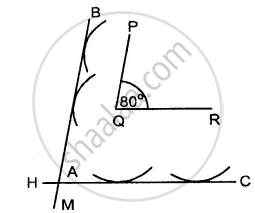# Construct an Angle Pqr = 80°. Draw a Line Parallel to Pq at a Distance of 3 Cm from It and Another Line Parallel to Qr at a Distance of 3.5 Cm from It. Mark the Point of Intersection - Mathematics

Sum

Construct an angle PQR = 80°. Draw a line parallel to PQ at a distance of 3 cm from it and another line parallel to QR at a distance of 3.5 cm from it. Mark the point of intersection of these parallel lines as A.

#### Solution

Steps of construction :1. Draw ∠PQR = 80°
2. With P as centre draw an arc of radius 2 cm.
3. Again with Q as a centre, draw another arc of radius 2 cm. Then BM is a line which touches the two arcs. Then BM is a line parallel to PQ.
4. With Q as a centre, draw an arc of radius 3.5 cm. With R as centre draw another arc of radius 3.5 cm. Draw a line HC which touches these two arcs. Let these two parallel lines intersect at A.
Concept: Construction of an Angle - Construction of Parallel Lines
Is there an error in this question or solution?
Chapter 18: Constructions - Exercise 18 (C) [Page 205]

#### APPEARS IN

Selina Concise Mathematics Class 8 ICSE
Chapter 18 Constructions
Exercise 18 (C) | Q 4 | Page 205
Share